# The Nets of Three Dimensional Shapes (Prisms and Pyramids) | Making Three Dimensional Shapes Using their Nets Primary 5 (Basic 5) Term 3 Week 5 Mathematics

### MATHEMATICS

THIRD TERM

WEEK 5

PRIMARY 5

THEME –  GEOMETRY

PREVIOUS LESSON – Meaning and Types of Angles | The Use of Parallel and Transversal Lines to Determine Corresponding Angles, Alternate Angles and Vertical Opposite Angles Term 3 Week 4 Mathematics

#### LEARNING AREA

1. Introduction

2. Meaning and Types of Three Dimensional Shapes

3. The Nets of Three Dimensional Shapes

4. Making Three Dimensional Shapes

5. Revision and Weekly Assessment (Test)

#### LEARNING OBJECTIVES

By the end of the lesson, most pupils should have attained the following objectives –

1. identify prisms and pyramids.

2. state properties of 3 dimensional shapes such as cubes, cuboids, pyramids, etc.

3. draw nets of 3-D shapes.

4. construct cube, Cuboid, and prisms.

5. Quantitative reasoning related to 3-dimensional shapes.

#### ENTRY BEHAVIOUR

The pupils can identify and describe shapes such as tin, cube of sugar and box (commonly known as carton)

#### INSTRUCTIONAL MATERIALS

The teacher will teach the lesson with the aid of chart showing three dimensional shapes and cardboard, pencil, ruler and gum.

#### METHOD OF TEACHING

Choose a suitable and appropriate methods for the lessons.

Note – Irrespective of choosing methods of teaching, always introduce an activities that will arouse pupil’s interest or lead them to the lessons.

#### REFERENCE MATERIALS

1. Scheme of Work

2. 9 – Years Basic Education Curriculum

3. Course Book

4. All Relevant Material

5. Online Information

### CONTENT OF THE LESSON

#### LESSON 1 – INTRODUCTION

ACTIVITY 1 – 2D AND 3D SHAPES

Three dimensional shapes are objects or shapes that three dimensions such as length, width, and height.

Three dimensional shapes are known as 3D shapes.

While two dimensional shapes have just two dimensions such as length and width.

Two dimensional shapes are also known as 2D shapes.

The properties of a three dimensional shapes are,

1. Faces

2. Edges

3. Vertices

ACTIVITY 2 – THREE DIMENSIONAL SHAPES

The following shapes are the basic three dimensional shapes we see around us,

1. Cube

2. Cuboid (Rectangular prism)

3. Triangular prism

4. Sphere

5. Cone

6. Cylinder

7. Pyramid

WORKING EXERCISE

Draw the following three dimensional shapes,

1. Cube

2. Cuboid (Rectangular prism)

3. Triangular prism

4. Sphere

5. Cone

6. Cylinder

7. Pyramid

#### LESSON 2 – THE NETS OF TRIANGULAR, SQUARE AND RECTANGULAR PRISMS

ACTIVITY 1 – NET OF THREE DIMENSIONAL

A net is a pattern made from two dimensional shape to form three dimensional shape.

THE NETS OF PRISM

The net of a prism consists of six faces.

Focus prisms are triangular, square and rectangular prisms.

NET OF TRIANGULAR PRISM

The net of a triangular prism consists of two triangle with three squares or rectangles.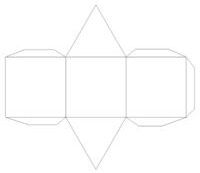NET OF SQUARE PRISM

The net of a square prism consists of six squares.NET RECTANGULAR PRISM

The net of a rectangular prism consists of two squares and four rectangles.

Rectangular prism is also as cuboid.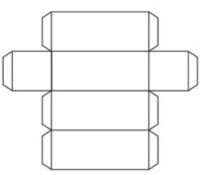ACTIVITY 2 – MAKING OF PRISM

Materials Needed are cardboard, pencil, ruler and gum.

Teacher organizes the pupils in groups or pairs depending on the size of the class and guides them,

1. to draw the nets of cube and cuboid.

2. use the nets to make cube and cuboid.

#### LESSON 3 – TRIANGULAR AND RECTANGULAR PYRAMIDS

ACTIVITY 1 – NETS OF SQUARE, TRIANGULAR AND RECTANGULAR PYRAMIDS

NET OF SQUARE PYRAMID

The net of a square pyramid consists of one square and four triangles.NET OF TRIANGULAR PYRAMID

The net of a triangles pyramid consists of four triangles.

One of the triangle is a base.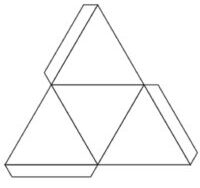NET OF RECTANGULAR BASE PYRAMIDS

The net of a rectangular pyramid consists of one rectangle and four triangles.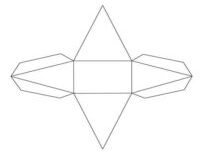ACTIVITY 2 – MAKING OF SQUARE, TRIANGULAR AND RECTANGULAR PYRAMIDS

Materials Needed are cardboard, pencil, ruler and gum.

Teacher organizes the pupils in groups or pairs depending on the size of the class and guides them,

1. to draw the nets of square, triangular and rectangular pyramids.

2. use the nets to make square, triangular and rectangular pyramids.

#### LESSON 4 – NET OF OTHER THREE DIMENSIONAL SHAPES

NET OF A CONE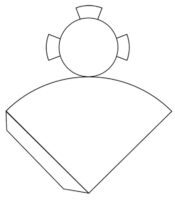NET OF A CYLINDER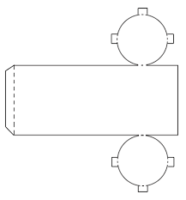MAKING OF A CONE AND CYLINDER

Teacher guides pupils as groups or pairs to make a cone and cylinder.

REVISION AND WEEKLY ASSESSMENT

As stated in performance objectives or lesson evaluation.

#### PRESENTATION

To deliver the lesson, the teacher adopts the following steps:

1. To introduce the lesson, the teacher revises the previous lesson. Based on this, he/she asks the pupils some questions;

2. Teacher organizes the pupils in groups or pair depending on the size of the class.

3. Teacher displays sample and chart of 2D and 3D shapes.

4. Teacher asks pupils as groups or pairs to identify and describe the shapes.

Pupil’s Activities – identify and describe the 2D and 3D shapes.

5. Teacher uses the sample, chart and pupil’s responses to introduce the lesson and leads a discussion on the nets of three dimensional shapes.

Pupil’s Activities – Pay attention to the lesson introduction to understand the concept of the lesson.

6. Teacher leads pupils as groups or pairs to draw the nets of different types of prisms and pyramids.

7. Teacher guides pupils to use the nets to make different types of prisms and pyramids.

Pupil’s Activities – Follow the instructions to draw the nets and make different types of prisms and pyramids.

8. Teacher summarizes the lesson on the board with appropriate evaluation.

Pupil’s Activities – Participate actively in the summary of the lesson and write as instructed.

#### CONCLUSION

To conclude the lesson for the week, the teacher revises the entire lesson and links it to the following week’s lesson.

#### NEXT LESSON

Measurement of Height and Distance | Conversion of Units in Height and Distance (Metres and kilometres) Primary 5 (Basic 5) Term 3 Week 6 Mathematics

#### LESSON EVALUATION

1. explain the meaning of 3 dimensional shapes.

2. differentiate between 2D and 3D shapes.

3. state the uses of three dimensional shapes.

4. state the properties of the following shapes,

• Cube
• Cuboid
• Triangular prism
• Cone
• Sphere
• Pyramid
• Cylinder

5. draw the net of the following:

• Square, triangular and rectangular prisms
• Square, triangular and rectangular pyramids

6. construct prism and pyramid.